# Dynamic Regions in Simple Chaotic Flows

J. C. Sprott
Department of Physics, University of Wisconsin, Madison, WI 53706, USA
April 27, 2013

In 1994 I proposed 14 simple 3-D chaotic flows with six terms and one quadratic nonlinearity, called Cases F - S: J. C. Sprott, Phys. Rev. E 50, R647-R650 (1994). Through linearly rescaling of the three variables and time, coefficients of four of the six terms can be made unity, which means that the systems are completely described by only two parameters. What follows are plots of the dynamic regions in this 2-D parameter space in which initial conditions are chosen from a Gaussian distribution of random numbers with mean zero and variance one, except that the unbounded regions are tested many times to try to find initial conditions that lead to an attractor. In the plots, red indicates stable equilibria (S), cyan indicates periodic limit cycles (P), black indicates chaotic strange attractors (C), and white indicates unbounded orbits (U). The original motivation of this study was to identify candidate cases that exhibit multistability (two coexisting attractors), which would be indicated by a dotted region in the plane. However, do not confuse embedded periodic windows in the chaotic regions or long duration transients with multistability. The best candidate for coexisting strange attractors and limit cycles appears to be case P.

 Case F:     dx/dt = y + z     dy/dt = -x + ay     dz/dt = x2 - bz Animated view of the strange attractor for a =  0.5, b = 1:Case G:     dx/dt = ax + bz     dy/dt = xz - y     dz/dt = -x + y Animated view of the strange attractor for a =  0.4, b = 1: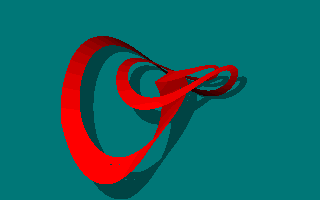Case H:     dx/dt = -y + z2         dy/dt = x + ay     dz/dt = x - bz Animated view of the strange attractor for a =  0.5, b = 1:Case I:     dx/dt = -ay     dy/dt = x + z     dz/dt = x + y2 - bz Animated view of the strange attractor for a =  0.2, b = 1: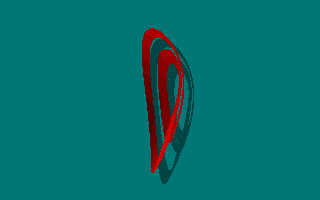Case J:     dx/dt = az     dy/dt = -by + z     dz/dt = -x + y + y2 Animated view of the strange attractor for a =  2, b = 2:Case K:     dx/dt = xy - z     dy/dt = x - y     dz/dt = ax + bz Animated view of the strange attractor for a =  1, b = 0.3:Case L:     dx/dt= y + az     dy/dt= 0.9x2 - y     dz/dt = b - x Animated view of the strange attractor for a =  3.9, b = 1:Case M:     dx/dt = -z     dy/dt = -x2 - y     dz/dt = a + bx + y Animated view of the strange attractor for a =  1.7, b = 1.7:Case N:     dx/dt = -ay     dy/dt = x + z2     dz/dt = 1 + y - bz Animated view of the strange attractor for a = 2, b = 2: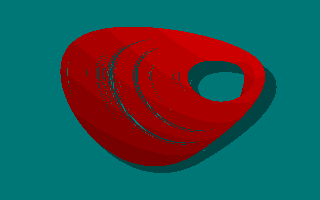Case O:     dx/dt = y     dy/dt = x - z     dz/dt = ax + xz + by Animated view of the strange attractor for a =  1, b = 2.7:Case P:     dx/dt = ay + z     dy/dt = -x + y2     dz/dt = x + by Animated view of the strange attractor for a =  2.7, b = 1: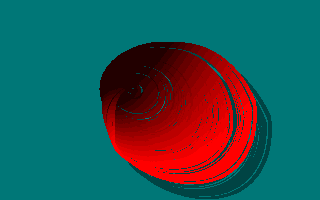Case Q:     dx/dt = -z     dy/dt = x - y     dz/dt = ax + y2 + bz Animated view of the strange attractor for a =  3.1, b = 0.5: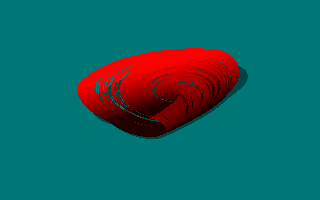Case R:     dx/dt = a - y     dy/dt = b + z     dz/dt = xy - z Animated view of the strange attractor for a =  0.9, b = 0.4:Case S:     dx/dt = -x - ay     dy/dt = x + z2     dz/dt = b + x Animated view of the strange attractor for a =  4, b = 1: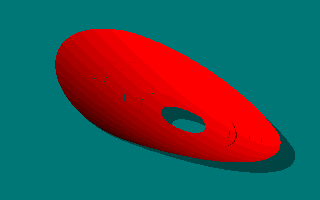Back to Sprott's Technical Notes